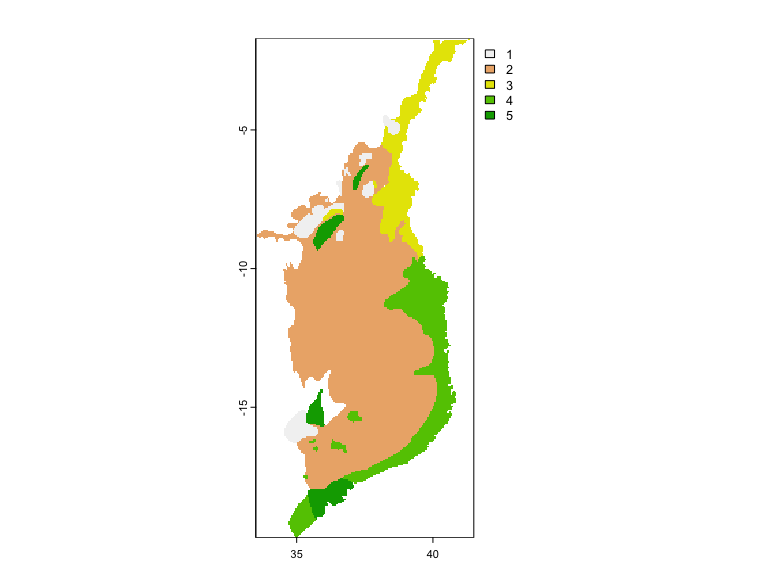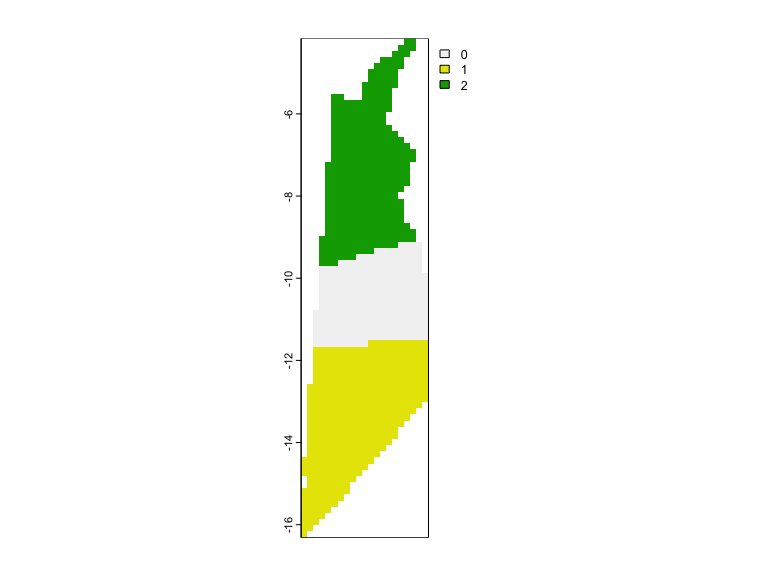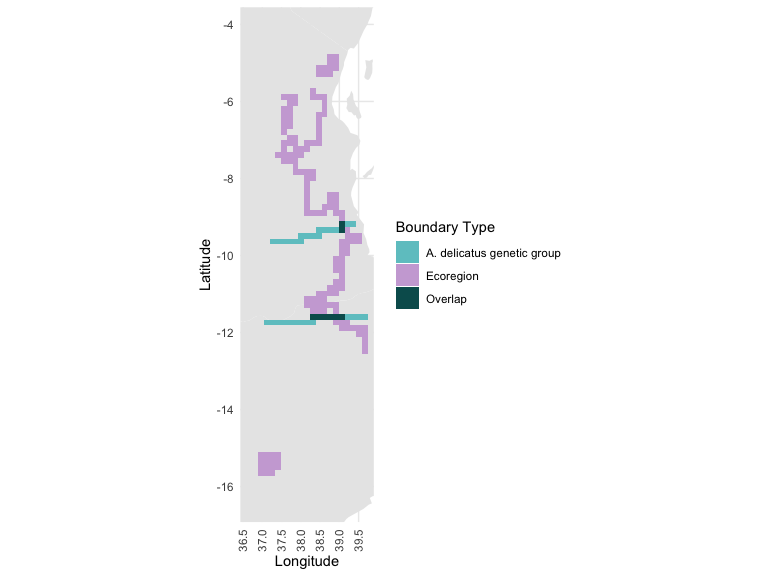# BoundaryStats Analysis Workflow

#### 2023-10-21

This vignette is a walkthrough of the workflow for calculating boundary statistics and boundary overlap statistics for two ecological variables. Here, we use East African ecoregion data and genomic analyses from Barratt et al. 2018. The following code tests for (1) whether there are significant geographic boundaries between genetic groups of the frog species Leptopelis flavomaculatus and (2) whether those boundaries overlap significantly with ecoregion boundaries.

## Load packages used in this vignette

library(BoundaryStats)
library(terra)
#> Warning: package 'terra' was built under R version 4.3.1
library(magrittr)

## Input data

### Ecoregion data

data(ecoregions)
ecoregions <- rast(ecoregions_matrix, crs = ecoregions_crs)
ext(ecoregions) <- ecoregions_ext
plot(ecoregions)### Genetic data

These data are based on the ADMIXTURE results from Barratt et al. 2018. The point data (assignment probabilities for each individual) have been interpolated using universal kriging to produce a raster surface.

data(L.flavomaculatus)
L.flavomaculatus <- rast(L.flavomaculatus_matrix, crs = L.flavomaculatus_crs)
ext(L.flavomaculatus) <- L.flavomaculatus_ext
plot(L.flavomaculatus)### Matching datasets

In order to test for significant overlap between the traits, the SpatRaster objects need to be aligned in extent, resolution, and projection. We are first matching the projection, then downsampling and cropping the ecoregion raster to match the genetic data. We are also masking the genetic raster with the ecoregion, since it originally includes space off the coastline.

crs(ecoregions) <- crs(L.flavomaculatus)
ecoregions <- resample(ecoregions, L.flavomaculatus) %>%
crop(., L.flavomaculatus) %>%
L.flavomaculatus <- crop(L.flavomaculatus, ecoregions) %>%
mask(., ecoregions)

## Define trait boundaries and ecoregion boundaries

There are two functions to define geographical boundaries in BoundaryStats, which take different data. The function define_boundary() takes either continuous trait data and boundary intensities. If inputting continuous trait data, use convert = T to convert from trait data into boundaries. If inputting boundary intensity data (e.g., urbanization metrics if urban land uses are boundaries), use convert = F to define boundaries based on an intensity threshold.

The two datasets in this vignette are categorical–ecoregion and genetic group identity–so we are using the other boundary definition function, categorical_boundary() to identify spatial transitions from one category to another.

ecoregions_boundaries <- categorical_boundary(ecoregions)
L.flavomaculatus_boundaries <- categorical_boundary(L.flavomaculatus)

## Plot boundary overlap

The overlap in boundaries between two variables can be plotted using the plot_boundary() function, which is a wrapper for ggplot2.

plot_boundary(L.flavomaculatus_boundaries, ecoregions_boundaries, trait_names = c('A. delicatus genetic group', 'Ecoregion'))## Calculate boundary statistics

### Create null distributions

The function boundary_null_distrib() simulates neutral landscapes based on the input data. The default number of iterations is 10, but a value between 100 and 1000 is recommended. This step may take a while, depending on the selected neutral model and number of iterations, so we are maintaining the default 10 iterations.

Three neutral models are currently available: complete stochasticity (default), Gaussian random fields, and modified random clusters. Random cluster models are suited to categorical variables like group identity (cat = T), so we use it here.

L.flav_bound.null <- boundary_null_distrib(L.flavomaculatus, cat = T, n_iterations = 10, model = 'random_cluster', p = 0.5, progress = F)
#> DONE

### Run statistical tests

n_subgraph is the number of subgraphs (i.e., contiguous groups of cells representing boundaries), and max_subgraph is the length of the longest subgraph.

n_subgraph(L.flavomaculatus_boundaries, L.flav_bound.null)
#>  n subgraphs      p-value
#> 2.0000000000 0.0002178985
max_subgraph(L.flavomaculatus_boundaries, L.flav_bound.null)
#> length of longest boundary                    p-value
#>               2.959129e+05               8.155239e-02

## Calculate boundary overlap statistics

### Create null distributions

Usage for overlap_null_distrib is similar to boundary_null_distrib, but takes raster surfaces for two traits (x and y), along with arguments for each trait. Since we are testing the effects of relatively static ecoregions on L. flavomaculatus population structure, we are not going to simulate randomized rasters for the ecoregions.

L.flav_overlap.null <- overlap_null_distrib(L.flavomaculatus, ecoregions, rand_both = F, x_cat = T, n_iterations = 10, x_model = 'random_cluster', px = 0.5, progress = F)
#> DONE

### Run statistical tests

Odirect is the number of directly overlapping boundary elements between the two variables. Ox is the average minimum distance for a a Trait x boundary element to the nearest Trait y boundary element. It assumes that boundaries for Trait x depend on the boundaries in Trait y. Oxy is the average minimum distance between boundary elements in x and y (x and y affect each other reciprocally).

Odirect(L.flavomaculatus_boundaries, ecoregions_boundaries, L.flav_overlap.null)
#> n overlapping boundary elements                         p-value
#>                       8.0000000                       0.1384157
Ox(L.flavomaculatus_boundaries, ecoregions_boundaries, L.flav_overlap.null)
#> average minimum distance (x depends on y)
#>                                  48465.28
#>                                   p-value
#>                                      0.00
Oxy(L.flavomaculatus_boundaries, ecoregions_boundaries, L.flav_overlap.null)
#> average minimum distance                  p-value
#>                 161879.4                      0.0

## Citation

Barratt, C.D., Bwong, B.A., Jehle, R., Liedtke, C.H., Nagel, P., Onstein, R.E., Portik, D.M., Streicher, J.W. & Loader, S.P. (2018) Vanishing refuge? Testing the forest refuge hypothesis in coastal East Africa using genome‐wide sequence data for seven amphibians. Molecular Ecology, 27, 4289-4308.